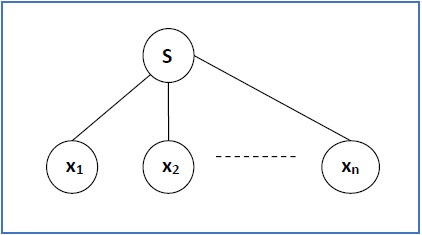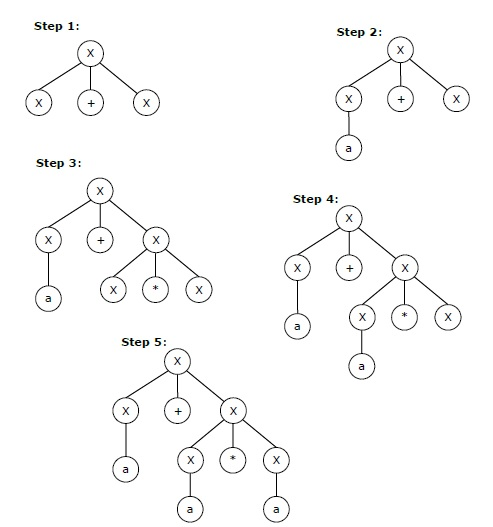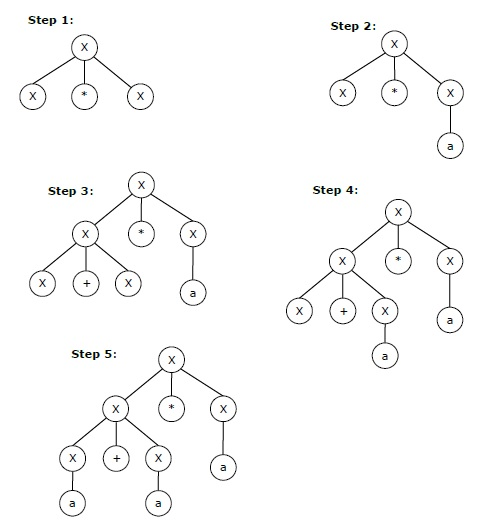# Context-Free Grammar Introduction

Definition − A context-free grammar (CFG) consisting of a finite set of grammar rules is a quadruple (N, T, P, S) where

• N is a set of non-terminal symbols.

• T is a set of terminals where N ∩ T = NULL.

• P is a set of rules, P: N → (N ∪ T)*, i.e., the left-hand side of the production rule P does have any right context or left context.

• S is the start symbol.

Example

• The grammar ({A}, {a, b, c}, P, A), P : A → aA, A → abc.
• The grammar ({S, a, b}, {a, b}, P, S), P: S → aSa, S → bSb, S → ε
• The grammar ({S, F}, {0, 1}, P, S), P: S → 00S | 11F, F → 00F | ε

## Generation of Derivation Tree

A derivation tree or parse tree is an ordered rooted tree that graphically represents the semantic information a string derived from a context-free grammar.

### Representation Technique

• Root vertex − Must be labeled by the start symbol.

• Vertex − Labeled by a non-terminal symbol.

• Leaves − Labeled by a terminal symbol or ε.

If S → x1x2 …… xn is a production rule in a CFG, then the parse tree / derivation tree will be as follows −There are two different approaches to draw a derivation tree −

Top-down Approach −

• Starts with the starting symbol S

• Goes down to tree leaves using productions

Bottom-up Approach −

• Starts from tree leaves

• Proceeds upward to the root which is the starting symbol S

### Derivation or Yield of a Tree

The derivation or the yield of a parse tree is the final string obtained by concatenating the labels of the leaves of the tree from left to right, ignoring the Nulls. However, if all the leaves are Null, derivation is Null.

Example

Let a CFG {N,T,P,S} be

N = {S}, T = {a, b}, Starting symbol = S, P = S → SS | aSb | ε

One derivation from the above CFG is “abaabb”

S → SS → aSbS → abS → abaSb → abaaSbb → abaabb### Sentential Form and Partial Derivation Tree

A partial derivation tree is a sub-tree of a derivation tree/parse tree such that either all of its children are in the sub-tree or none of them are in the sub-tree.

Example

If in any CFG the productions are −

S → AB, A → aaA | ε, B → Bb| ε

the partial derivation tree can be the following −If a partial derivation tree contains the root S, it is called a sentential form. The above sub-tree is also in sentential form.

### Leftmost and Rightmost Derivation of a String

• Leftmost derivation − A leftmost derivation is obtained by applying production to the leftmost variable in each step.

• Rightmost derivation − A rightmost derivation is obtained by applying production to the rightmost variable in each step.

Example

Let any set of production rules in a CFG be

X → X+X | X*X |X| a

over an alphabet {a}.

The leftmost derivation for the string "a+a*a" may be −

X → X+X → a+X → a + X*X → a+a*X → a+a*a

The stepwise derivation of the above string is shown as below −The rightmost derivation for the above string "a+a*a" may be −

X → X*X → X*a → X+X*a → X+a*a → a+a*a

The stepwise derivation of the above string is shown as below −## Left and Right Recursive Grammars

In a context-free grammar G, if there is a production in the form X → Xa where X is a non-terminal and ‘a’ is a string of terminals, it is called a left recursive production. The grammar having a left recursive production is called a left recursive grammar.

And if in a context-free grammar G, if there is a production is in the form X → aX where X is a non-terminal and ‘a’ is a string of terminals, it is called a right recursive production. The grammar having a right recursive production is called a right recursive grammar.